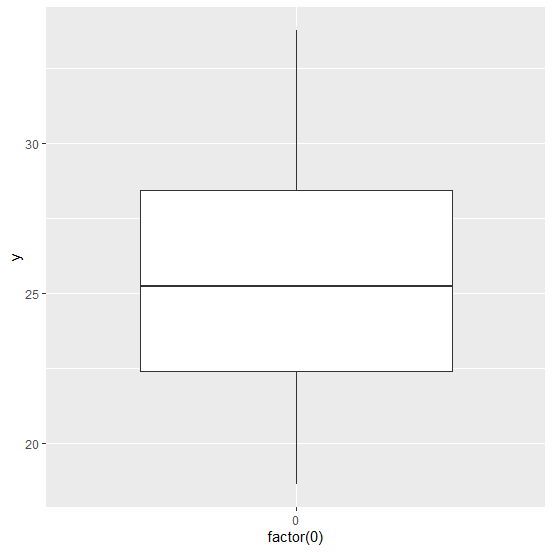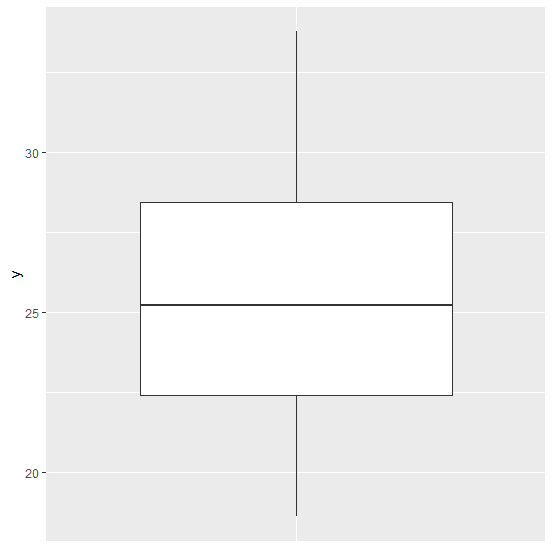# How to create a boxplot using ggplot2 for single variable without X-axis labels in R?

The important part of a boxplot is Y−axis because it helps to understand the variability in the data and hence, we can remove X−axis labels if we know the data description. To create a boxplot using ggplot2 for single variable without X−axis labels, we can use theme function and set the X−axis labels to blank as shown in the below example.

## Example

Live Demo

Consider the below data frame −

y<−rnorm(20,25,4.2)
df<−data.frame(y)
df

## Output

y
1 30.52520
2 23.95832
3 25.47747
4 28.13632
5 33.78174
6 18.61764
7 20.46791
8 29.65309
9 22.46586
10 19.87244
11 22.32916
12 26.26577
13 30.54542
14 27.85693
15 23.60995
16 19.79125
17 27.01937
18 22.43575
19 29.34608
20 24.97311

## Example

library(ggplot2)
ggplot(df,aes(x=factor(0),y))+geom_boxplot()

## OutputCreating the boxplot without X-axis labels −

## Example

ggplot(df,aes(x=factor(0),y))+geom_boxplot()+theme(axis.title.x=element_blank(),axis.text.x=element_blank(),axis.ticks.x=element_blank())

## Output